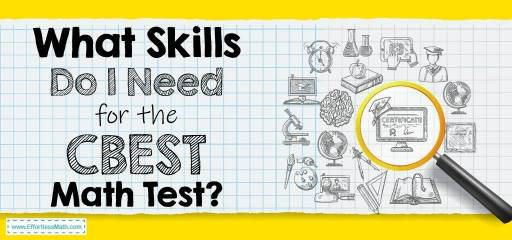# What Skills Do I Need for The CBEST Math Test?As a potential new teacher in the California public school system, you must pass the California Basic Educational Skills Test (CBEST).

This test is divided into multiple sections, and one of the hardest is the math test which you will need to pass.

Below is a guide to help you understand the test and what skills you will need to pass the CBEST math test.

The Absolute Best Book to Ace the CBEST Math Test

## CBEST Math Test Format

The test can be taken three ways:

• As a computer-based test at a testing center.
• As an online proctored test, which can be performed from your home or office.
• Paper tests can be conducted at a testing center. However, this option is being removed.

If you intend to take a paper-based test, you will have a total of four hours to complete the math and other sections of the test. The tests use a combined time, and you need to ensure you manage your time effectively.

The computer-based CBEST allows two hours for the math section to be completed. When performed on a computer, each test is administered in different rooms, so you can’t go back and forth on different tests.

The math section will consist of 50 multiple choice questions with five possible answers.

You cannot use a calculator during your test, and you cannot bring a smartphone or other device in either.

A Comprehensive Bundle to Help you prepare for the CBEST Math Test

## Math Skills Required to Pass the CBEST Math Test

The CBEST math test will not only test your understanding and ability to solve problems, but it will also test your ability to apply mathematical knowledge into a teaching environment.

Most of the math being tested in the CBEST is high school level because that is what you’ll be teaching. However, you won’t need high-level or college math understanding to pass the test.

There are three critical skill areas you will need to understand and use to pass the CBEST math test. Each area will have multiple ideas and principles you will need to understand.

### Estimation, Measurement, and Statistical Principles

The Estimation, measurement, and statistical principles areas will be testing your understanding of statistical properties and functions. You will also need the ability to estimate numerical and measurement-based data.

The questions in this section are broken down even further into:

#### Statistical Principles

These questions will test your knowledge of probability, percentiles, and averages. You will also be tested on basic elements of statistics.

Some examples of things you’ll need to know are:

• Use data to find the average, proportion, or percentage.
• How to predict an outcome based on probability.
• How to compare exam results between students in a classroom.

#### Estimation and Measurement

These questions will test your knowledge of numerical guessing. You will need to estimate answers for arithmetic equations. Find measurements under specific conditions or contexts. Analyze word problems with time-sensitive situations.

Some examples of things you’ll need to know are:

• Estimate the amount of time it will take to complete something
• Estimate the result of a problem before calculating it
• How to measure length and perimeter for various geometric figure
• Standard measurements for length, temperature, weight, and capacity

### Computation and Problem Solving

These questions will test your understanding of basic arithmetic functions, such as division, multiplication, subtraction, and addition. Some questions will be in the form of word problems.

Some examples of things you’ll need to know are:

• How to use arithmetic functions with whole numbers and add or subtract positive and negative numbers.
• How to solve practical math problems such as determine a total based on unit price.
• How to solve problems when there is one unknown
• How to recognize problems without enough information, or if there are multiple options to solve a problem.

### Numerical and Graphical Relationships

These questions will test your understanding of graphs, charts, spreadsheets, tables, and percentages.

• How to organize numbers on a number line.
• How to use greater than, less than, equal to, and other mathematical symbols.
• How to identify mathematically equivalent numbers, expressions, and formulas.
• How to apply logical connectives (if/then) and quantifiers (some, all, none) to solve problems.
• How to use tables, spreadsheets, and graphs (circle, bar, and line) to solve problems

## Final Thoughts

The CBEST is a critical test to ensure you are ready to teach high school students. The test is not easy, but you will do it with the proper study and practice.

Make sure you get a good study guide, use online practice tests, and focus on areas you are struggling with.

The Best Book to Ace the CBEST Test

## More from Effortless Math for CBEST Test …

### Don’t know the best study guides for CBEST math self-study?

5 Best CBEST Math Study Guides Provides you with complete information about the best.

### Do you intend to become a teacher by passing the CBEST test?

Maybe this is your mental question: Do you Need to Pass the CBEST to be a Substitute Teacher?

### Is your CBEST math test approaching and you are anxious about it?

We understand your concerns and are committed to addressing them: Top 10 Tips to Overcome CBEST Math Anxiety

The Perfect Prep Books for the CBEST Math Test

## Have any questions about the CBEST Test?

### What people say about "What Skills Do I Need for The CBEST Math Test? - Effortless Math: We Help Students Learn to LOVE Mathematics"?

No one replied yet.

X
49% OFF

Limited time only!

Save Over 49%

SAVE \$34

It was \$69.99 now it is \$35.99